## SOLVED: Normal Distribution & Sampling Distribution (TiNspire)

Below is a typical Normal Distribution problem that will be solved further below using the Statistics Made Easy app .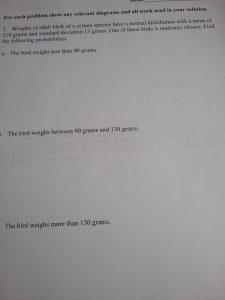Using the Normal Distribution feature in the Statistics Made Easy app , we enter the given mean and standard deviation in the top box use 0 and 19 for A and B to 0.0324 or 3.24% .For question 2 we use 90 and 130 instead in the 2. box to 85.83%For the 3. question we use 130 as A and some large B such as 500 (or 1000) to get 0.1092 or 10.92% as the answer.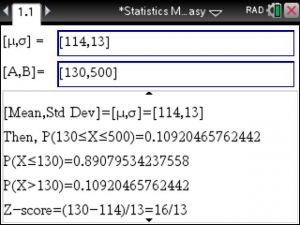For Sampling Distribution we use the Central Limit Theorem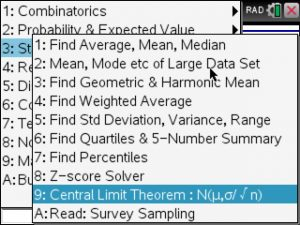and enter the known mean, standard deviation and sample size to get the sampling distribution

N(mean, std dev/square root(n) ). Here we get 114 and 1.3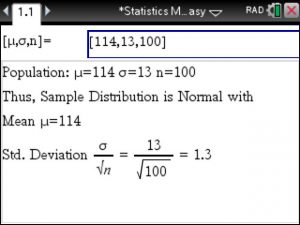Since the sampling distribution is a normal distribution , we go to the previous and only change the standard deviation to 1.3 instead of 13. Below, we type in the given 110 and 116 to get 93.6986%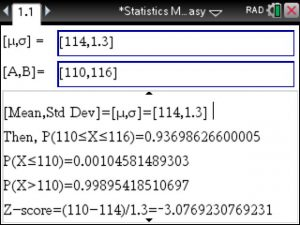That was not too difficult at all! It was easy..

Posted on Categories statistics, tinspirecx

## Confidence Interval for Proportions using the exact Bliss Method on the TiNspire

When finding Confidence Intervals for Proportions we typically involve the Normal Distribution to create an confidence Interval estimate.

However, this method requires the conditions np<5 and n(1-p)<5 to be fulfilled.

It is not uncommon to have a large sample size n available which likely disables this method.

This is where the Bliss Method comes in to find the exact Confidence Interval on the TiNspire.